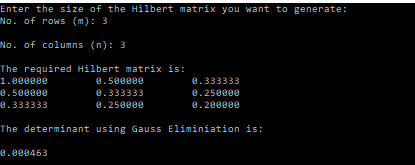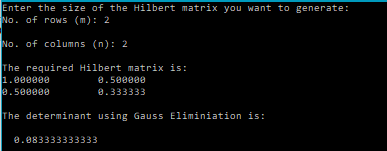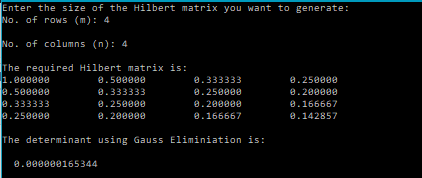# Determinant of the Hilbert Matrix – C PROGRAM

I have recently written a post that calculates the determinant of a given square matrix using the Gaussian elimination technique. In the last post I wrote about generating Hilbert matrices using C programming.

In this post we extend that mix the two ideas to evaluate the determinants of the Hilbert matrices for various orders. The wolfram mathworld page has already listed the determinants for the first 6 orders, so we have a way to check if our code is correct or not.

### CODE:

```/************************************
****DETERMINANT OF HILBERT MATRIX****
************************************/
#include<stdio.h>
#include<math.h>
/*******
Function that calculates the determinant of a square matrix using Gauss-Elimination :
Pass the square matrix as a parameter, and calculate and return the dete
Parameters: order(n),matrix[n][n]
********/
double determinant(int n, double a[n][n]){
double det=1;
int i;
int swapCount=gaussElimination(n,n,a);
for(i=0;i<n;i++){
det =det*a[i][i];
}
return det*pow(-1,swapCount);
}
/********
Function that perform Gauss Elimination
Pass the square matrix as a parameter, and calculate and store the upperTriangular(Gauss-Eliminated Matrix) in it
Parameters: rows(m),columns(n),matrix[m][n]
********/
int gaussElimination(int m, int n, double a[m][n]){
int i,j,k;
int swapCount=0;
for(i=0;i<m-1;i++){
//Partial Pivoting
for(k=i+1;k<m;k++){
//If diagonal element(absolute vallue) is smaller than any of the terms below it
if(fabs(a[i][i])<fabs(a[k][i])){
//Swap the rows
swapCount++;
for(j=0;j<n;j++){
double temp;
temp=a[i][j];
a[i][j]=a[k][j];
a[k][j]=temp;
}
}
}
//Begin Gauss Elimination
for(k=i+1;k<m;k++){
double  term=a[k][i]/ a[i][i];
for(j=0;j<n;j++){
a[k][j]=a[k][j]-term*a[i][j];
}
}
}
return swapCount;
}
/*******
Function that reads the elements of a matrix row-wise
Parameters: rows(m),columns(n),matrix[m][n]
*******/
void readMatrix(int m, int n, double matrix[m][n]){
int i,j;
for(i=0;i<m;i++){
for(j=0;j<n;j++){
scanf("%lf",&matrix[i][j]);
}
}
}
/*******
Function that prints the elements of a matrix row-wise
Parameters: rows(m),columns(n),matrix[m][n]
*******/
void printMatrix(int m, int n, double matrix[m][n]){
int i,j;
for(i=0;i<m;i++){
for(j=0;j<n;j++){
printf("%lf\t",matrix[i][j]);
}
printf("\n");
}
}
/*******
Function that copies the elements of a matrix to another matrix
Parameters: rows(m),columns(n),matrix1[m][n] , matrix2[m][n]
*******/
void copyMatrix(int m, int n, double matrix1[m][n], double matrix2[m][n]){
int i,j;
for(i=0;i<m;i++){
for(j=0;j<n;j++){
matrix2[i][j]=matrix1[i][j];
}
}
}
/********
Function that generates a Hilbert matrix
Parameters:
no. of rows: m,
no. of coulmns: n,
a matrix of size mxn that would store the Hilbert matrix
********/
void Hilbert(int m, int n, double H[m][n]){
int i,j;
for(i=0;i<m;i++){
for(j=0;j<n;j++){
H[i][j]=(double)1.0/((i+1)+(j+1)-1.0);
}
}
}
int main(){
int m,n,i,j;
printf("Enter the size of the Hilbert matrix you want to generate:\nNo. of rows (m): ");
scanf("%d",&m);
printf("\nNo. of columns (n): ");
scanf("%d",&n);
double H[m][n];
Hilbert(m,n,H);
printf("\nThe required Hilbert matrix is:\n");
printMatrix(m,n,H);
printf("\nThe determinant using Gauss Eliminiation is:\n\n%16.12lf\n",determinant(n,H));

}
```

### OUTPUT:### References and Resources:

http://mathworld.wolfram.com/HilbertMatrix.html

[wpedon id="7041" align="center"]

This site uses Akismet to reduce spam. Learn how your comment data is processed.